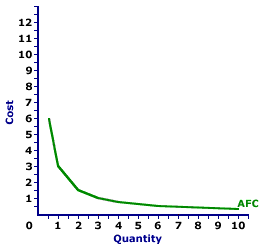Sunday  February 5, 2023
 AmosWEB means Economics with a Touch of Whimsy!DISINVESTMENT: A drop in the total quantity of capital in the economy because the depreciation of existing capital is greater than investment in new capital. In other words, the capital we have is wearing out faster than we're replacing it with new stuff. This isn't good. At best, it limits economic growth and might even cause the economy's pie to shrink if increases in other resources don't kick in.AVERAGE FIXED COST CURVE:

A curve that graphically represents the relation between average fixed cost incurred by a firm in the short-run product of a good or service and the quantity produced. This curve is constructed to capture the relation between average fixed cost and the level of output, holding other variables, like technology and resource prices, constant. The average fixed cost curve is one of three average curves. The other two are average total cost curve and average variable cost curve. A related curve is the marginal cost curve.
Average Fixed Cost CurveThe average fixed cost curve is negatively sloped. Average fixed cost is relatively high at small quantities of output, then declines as production increases. The more production increases, the more average fixed cost declines. The reason behind this perpetual decline is that a given FIXED cost is spread over an increasingly larger quantity of output.

The graph to the right is the average fixed cost curve for the short-run production of Wacky Willy Stuffed Amigos (those cute and cuddly armadillos and tarantulas). The quantity of Stuffed Amigos production, measured on the horizontal axis, ranges from 0 to 10 and the average fixed cost incurred in the production of Stuffed Amigos, measured on the vertical axis, ranges from a high of \$6 to a low of \$0.30. Actually, if the quantity is extended beyond 10 Stuffed Amigos, then average fixed cost is less than \$0.30. Or if the quantity is reduced below 1/2 unit, then average fixed cost is greater than \$6. For the geometrically inclined, this average fixed cost curve is a rectangular hyperbola.

This declining average fixed cost curve is a major reason that the average total curve is negatively sloped for relatively small output quantities. In fact, firms that use a lot of fixed inputs relative to variable inputs, such that fixed cost is a substantial share of total cost, spend a lot of their production time in the decreasing portion of the average total cost curve. This has a big impact on how these firms operate. If average total cost declines with additional production, then a firm can profitably charge a lower price with increased output.

 <= AVERAGE FIXED COST AVERAGE-MARGINAL RELATION =>Recommended Citation:

AVERAGE FIXED COST CURVE, AmosWEB Encyclonomic WEB*pedia, http://www.AmosWEB.com, AmosWEB LLC, 2000-2023. [Accessed: February 5, 2023].

Check Out These Related Terms...

Or For A Little Background...

And For Further Study...
Search Again?PURPLE SMARPHIN[What's This?] Today, you are likely to spend a great deal of time lost in your local discount super center hoping to buy either looseleaf notebook paper or a three-hole paper punch. Be on the lookout for florescent light bulbs that hum folk songs from the sixties.Your Complete ScopeLewis Carroll, the author of Alice in Wonderland, was the pseudonym of Charles Dodgson, an accomplished mathematician and economist."Live in such a way that you would not be ashamed to sell your parrot to the town gossip."-- Will RogersMCAMonetary Control Act of 1980A PEDestrian's Guide Xtra CreditTell us what you think about AmosWEB. Like what you see? Have suggestions for improvements? Let us know. Click the User Feedback link.| | | | | | | | | | |
| | | |

Thanks for visiting AmosWEB